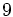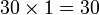# Groups of order 1536

## Contents

See pages on algebraic structures of order 1536| See pages on groups of a particular order

## Statistics at a glance

The number 1536 has prime factorization$1536 = 3 \cdot 2^9$. Because the order has only two distinct prime factors, and order has only two prime factors implies solvable, all groups of this order are solvable groups.

Quantity Value Explanation
Number of groups up to isomorphism 408641062
Number of abelian group up to isomorphism 30 (number of abelian groups of order$2^9$) times (number of abelian groups of order$3^1$) = (number of unordered integer partitions of$9$) times (number of unordered integer partitions of$1$) =$30 \times 1 = 30$
Number of nilpotent groups up to isomorphism 10494213 (number of groups of order 512) times (number of groups of order 3) =$10494213 \times 1 = 10494213$
Number of solvable groups up to isomorphism 408641062 Because the order has only two distinct prime factors, and order has only two prime factors implies solvable, all groups of this order are solvable groups.

## GAP implementation

The order 1536 is part of GAP's SmallGroup library. Hence, any group of order 1536 can be constructed using the SmallGroup function by specifying its group ID. Unfortunately, IdGroup is not available for this order, i.e., given a group of this order, it is not possible to directly query GAP to find its GAP ID.

Further, the collection of all groups of order 1536 can be accessed as a list using GAP's AllSmallGroups function. However, the list size may be too large relative to the memory allocation given in typical GAP installations. To overcome this problem, use the IdsOfAllSmallGroups function which stores and manipulates only the group IDs, not the groups themselves.

Here is GAP's summary information about how it stores groups of this order, accessed using GAP's SmallGroupsInformation function:

gap> SmallGroupsInformation(1536);

There are 408641062 groups of order 1536.
1 - 10494213 are the nilpotent groups.
10494214 - 408526597 have a normal Sylow 3-subgroup.
408526598 - 408544625 have a normal Sylow 2-subgroup.
408544626 - 408641062 have no normal Sylow subgroup.

This size belongs to layer 8 of the SmallGroups library.
IdSmallGroup is not available for this size.